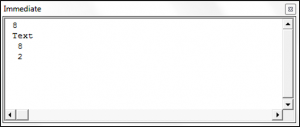# VBA Convert Integer (Number) to String

We have already covered an introduction to string functions in our VBA Strings and Substrings Functions tutorial. In this tutorial, we are going to look at how to convert an integer to a string (click here to learn about converting Strings to Numbers). The reason you would want to convert a number or date to a string is in order to use string manipulation functions on these values.

### The VBA CStr Function

The VBA CStr Function allows you to convert a number, date or boolean data type to a string.

The syntax of the CStr Function is:

CStr(expression) where expression is the number or date that you want to convert.

The following code shows you how numbers are outputted compared to text, including by using the CStr Function.Both CStr(8) and the word Text are displayed as text and are left aligned whereas the two numbers are right aligned within the immediate window.